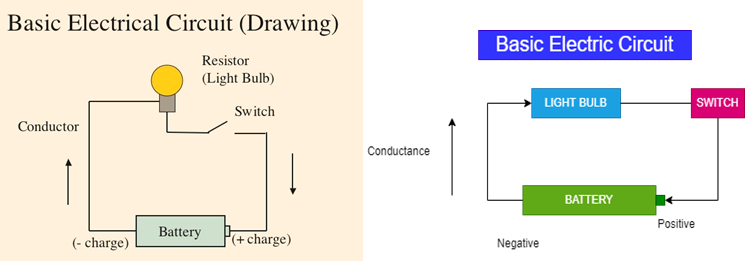# Difference between the electric circuit and magnetic circuit## Explain about the Electric circuit

A simple electric circuit consists of a voltage source, some kind of load, and a conductor between the voltage source and the load to allow the flow of electrons. There is a fundamental relationship exists between current, voltage, and resistance in an electric circuit. In the following circuit, a battery acts as the voltage source, an electrical wire is used for the conductor, and light acts as a load. A switch has been introduced into this circuit. Always there should be a complete path for current to flow. If the switch is open, the path is incomplete and the light will not illuminate. Closing the switch completes the path, allowing electrons to leave the negative terminal and flow through the light to the positive terminal, shown in the figure below.

## Schematic Diagram

The following schematic is a representation of an electrical Schematic circuit, consisting of a cell, a resistance, a voltmeter, and an ammeter. The ammeter is connected in series with the circuit, this will indicate the amount of current flow in the circuit. The voltmeter connected across the resistance will show the value of the voltage supplied to the load.

### Ohms law of Electric circuit:

Ohms law states that current through a conductor between two points is directly proportional to the voltage across those two points.

V=Voltage, I=current, R =Resistance

V=I * R

I=V/R

R=V/I

## What is meant by Magnetism?

A substance is said to be a magnet if it has a property of magnetism that means if it has the power to attract substances such as Iron, Steel, Nickel, or Cobalt known as magnetic material.

The point of maximum attraction is called magnetic poles, all magnets have at least two poles. The point that faces toward northward is called the north pole and the point face toward the southward is called a south pole.

Various characteristics of magnetic lines of force can be described as follows

1. Magnetic lines of force are continuous and will always form closed loops.
2. Magnetic lines of force will never cross one another.
3. Parallel magnetic lines of force traveling in the same direction repel one another,
4. Parallel magnetic lines of force traveling in opposite directions tend to unite with each other and form into single lines traveling in a direction determined by the magnetic poles creating the lines of force.
5. The magnetic lines of force existing between two unlike poles cause the pole to be pulled together.
6. Magnetic lines of force pass through all materials, both magnetic and Nonmagnetic.

## What is meant by Magnetic Field?

The space surrounding a magnet in which the magnetic force acts is called Magnetic Field.

Michael Faraday visualized the magnetic Field as being in a state of stress and consisting of a uniformly distributed line of force.

### What is meant by Magnetic Flux?

The Entire quantity of magnetic lines surrounding a magnet is called Magnetic flux. Flux in a magnetic circuit can be directly compared  to current in an electric circuit

### What is meant by Magnetic Flux Density?

The number of lines of force per unit area is called flux density and is measured in line per square Inch or lines per square centimeter

B=Flux.

ɸ= Total number of lines of Flux.

A=cross sectional area of the magnetic Circuit. B   = ɸ/A

### What is meant by the Magnetomotive force?

The ability of a coil to produce magnetic flux is called Magnetomotive force.

The amount of flux density in a core will depend upon five factors- The Current, Number of turns, Material of the magnetic core, Length of the core, and Cross-sectional area of the core.

More current and the more turns of wire we use, the greater will be the magnetizing effect.

MMF =N*I ampere-turns

N=Number of turns in the coil.

I=Electric Current through the circuit

### What is meant by Magnetic Field intensity?

When the number of turns of wire carrying current, the MMF created in the circuit is Ni ampere turns with l representing the mean path length for the magnetic flux, the magnetic field intensity is

Magnetic Field Intensity H = Ni/l ampere-turns/meter.

B= µ H

### What is meant by Magnetic Circuit?

The magnetic circuit is nothing but the path of magnetic flux, the MMF of the circuit creates flux in the path against the reluctance of the path.

Flux = mmf/Reluctance

### Ohms law of Magnetic circuit

The MMF of a magnetic circuit is directly proportional to flux established in it provided no part of the magnetic circuit is saturated, the constant of proportionality is the reluctance of the magnetic circuit   MMF α flux or MMF = Flux X Reluctance